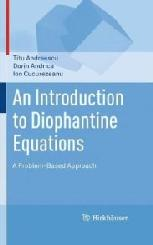## An Introduction to Diophantine Equations: A Problem-Based Approach Titu Andreescu Dorin Andrica Ion CucurezeanuDiophantus' Arithmetica is a collection of problems each followed by a solution. My first thought on picking up a book with the subtitle "A Problem-Based Approach" was that this is quite an appropriate way to treat the Diophantine equations: to follow in the footsteps of Diophantus himself. But the book actually goes beyond the classic format. The statements in the book are of the three sorts: theorems, examples, and exercises and problems. All come with solutions (proofs for the theorems) in the spirit of Diophantus, but the distinction makes it easier for a student to grasp the essential followed up by the first applications and then the means to verify his/her understanding of the material. The book also contains some historical information on Diophantus himself (in Preface), occasional remarks, while, in case of Pell's equations, a whole section in the third chapter offers a historic sketch of the treatment of quadratic equations in integers.

The book consists of two parts. The authors claim (viii) that there are three chapters in the first part. In fact there are four. This is the most amusing typo in a book on solving equations in integers. Chapters are subdivided into topical sections. At the end of each section there are numerous problems whose solutions are collected in Part II of the book that, naturally enough, also consists of four chapters. Many examples and exercises come from various national and international olympiads; some are original (as are many solutions) with the authors.

Diophantus' preface to his Arithmetica is very suitable for the book at hand (I. Thomas, Greek Mathematical Works I):

Now let us tread the path to the propositions themselves, which contain a great mass of material comprised into various species. As they are both numerous and very complex to express, they are only slowly grasped by those into whose hands they are put, and include things hard to remember; for this reason I have tried to divide them up according to their subject-matter, and especially to place, as is fitting, the elementary propositions at the beginning in order that passage may be made from the simpler to the more complex.

Chapter 1 is introductory where the authors highlight several elementary methods for solving Diophantine equations. The main among these are the factoring, the parametric method, modular arithmetic, induction, and infinite descent. Chapter 2 deals with the classical equations: linear and Pythagorean in the first two sections, with additional examples in the third section. Chapter 3 is devoted to Pell's equations. Chapter 4 covers advanced methods, like Gaussian integers, rings Q[d], quadratic reciprocity.

Diophantus is deservedly considered and is so referred to in the book, as the "father of algebra". Millennia has passed since Diophantus had pioneered special notations for mathematical objects. He may have been understandably apprehensive whether his symbolism for the propositions that were "very complex to express" will be well received. The book at hand is intended for high school students, undergraduates and math teachers. It is written in the language that every one in these groups must be familiar with. The exposition is very lucid and the proofs are clear and instructive. The book will be an invaluable source for math contests participants and other math fans. It will be an excellent addition to any math library.An Introduction to Diophantine Equations: A Problem-Based Approach, Birkhäuser, 2010, 345 pp, \$59.95. ISBN 0817645489.